NCERT Class 12 Chemistry Solutions for Chapter 9 Coordination Compounds provides in-depth knowledge of the concepts given in the chapter. It helps the students prepare well for boards as well as competitive exams. It also helps the students strengthen their basics for advanced concepts.

The students appearing for UP board, MP board, CBSE, Gujarat board, Maharashtra board, etc. can refer to these NCERT Solutions and score well in the examination.

 Board CBSE Textbook NCERT Class Class 12 Subject Chemistry Chapter Chapter 9 Chapter Name Coordination Compounds Number of Questions Solved 43 Category NCERT Solutions

## NCERT Solutions for Class 12 Chemistry Chapter 9 Coordination Compounds

Complex compounds are known as coordination compounds. It is an important chapter from the examination perspective. The students need to be thorough with the concepts such as properties, examples and applications of coordination compounds.

A thorough knowledge of the basic concepts helps the students while studying the advanced concepts. This not only helps them during boards and home exams but also during competitive exams.

NCERT IN-TEXT QUESTIONS

Question 1.
Write the formulas of the following coordination compounds:
(a) tetraamminediaquacobalt (III) chloride
(b) potassium tetracyanonickelate (II)
(c) tris (ethane-1, 2-diamine) chromium (III) chloride
(d) amminebromidochloridonitrito-N-platinate (II)
(e) dichlorobis (ethane-1, 2-diamine) platinum (TV) nitrate
(f) iron (III) hexacyanoferrate (II).
(a) [Co(NH3)4(H2O)2]Cl3
(b) K2[Ni(CN)4]
(c) [Cr(en)3]Cl3
(d) [Pt(NH3)BrCl(NO2)]
(e) [PtCl2(en)2](NO3)2
(f) Fe4[Fe(CN)6]3.

Question 2.
Write the IUPAC names of the following coordination compounds:

1. [Co(NH3)6]Cl3
2. [Co(NH3)5Cl]Cl2
3. K3[Fe(CN)6l
4. K3lFe(C2O4)3]
5. K2[PdCl4]
6. [Pt(NH3)2Cl(NH2CH3)]Cl

1. Hexaamminecobalt (III) chloride
2. Pentaamminechloridecobalt (III) chloride
3. Potassium hexacyanoferrate (III)
4. Potassiumtrioxalatoferrate(III)
6. Diamminechloride (methylamine) platinum (II) chloride

Question 3.
Give the types of isomerism exhibited by the following complexes and draw the structures of these isomers:
(a) K[Cr(H2O)2(C2O4)2]
(b) [Co(en)3]Cl3
(c) [CO(NH3)5(NO2)] (NO3)2
(d) [Pt(NH3) (H2O)Cl2] (C.B.S.E. Outside Delhi 2013)
(a) K[Cr(H2O)2(C2O4)2] or K[Cr(H2O)2(OX)2]
(i) It exists as geometrical isomers :(ii) The cis isomer can also exist as pair of optical isomers.(b) The complex can exist as optical isomers. For structure, consult section 9.7.
(c) The complex can exist as pair of ionisation isomers as well as linkage isomers.
Ionisation isomers: [Co(NH3)5(NO2)] (NO3)2 and [Co(NH3)5(NO3)](NO2)(NO3)
(d) The complex can exist as pair of geometrical isomers.Question 4.
Give evidence to show that [Co(NH3)5Cl]SO4 and [CO(NH3)5SO4]Cl exist as ionisation isomers.
Dissolve both the complexes separately in water. First add a few drops of BaCl2 solution to both these complexes. Only one of these will give white precipitate with the solution indicating that SO4 is not a part of complex entity. If exists as an anion.Now again add a few drops of AgN03 solution to both these complexes. Only one of these will give white precipitate with the solution indicating that in this case Cl is not a part of complex entity. It exists as anion.This shows that the complexes exist as pair of ionisation isomers.

Question 5.
Explain on the basis of valence bond theory that [Ni(CN)4]2- ion with square planar structure is diamagnetic and the[Ni(CN)4]2- ion with tetrahedral geometry is paramagnetic.Question 6.
[MCl4]2- is paramagnetic while [Ni(CO)4] is diamagnetic though both are tetrahedral. Why ? (C.B.S.E. Outside Delhi2012)
In the complex [NiCl4]2-, Ni is in + 2 oxidation state and has the configuration 3d840. The CL ion being a weak ligand cannot pair the two unpaired electrons present in 3d orbitals. This means that 3d orbitals are not involved in hybridisation. The complex is sp3 hybridised (tetrahedral) and is paramagnetic in nature. In the other complex [Ni(CO)4], the oxidation state of Ni is zero and electronic configuration is 3d84s2. In the presence of the ligand CO, the 4s electrons shift to the two half filled 3d orbitals and make all the electrons paired. The valence 4s and 3p orbitals are involved in hybridisation. The complex is tetrahedral but diamagnetic. For more details, consult text part.

Question 7.
[Fe(H2O)6]3+ is strongly paramagnetic whereas [Fe(CN)6]3- is weakly paramagnetic. Explain.
Outer electronic configuration of iron (Z = 26) in-ground state is 3d64s2. Iron in this complex is in +3 oxidation state. Iron achieves +3 oxidation state by the loss of two 4s electrons and one 3d electron. The resulting Fe3+ ion has outer electronic configuration of 3d5. Since H2O is not a strong field ligand, it is unable to cause electron pairing.

Outer electronic configuration of iron (Z=26) in ground state is 3d64s2. Iron in this complex is in a +3 oxidation state. Iron achieves +3 oxidation state by the loss of two 4s electrons and one 3d electron. The resulting Fe3+ ion has an outer electronic configuration as 3d5. CN ion is a strong field ligand

Question 8.
Explain [Co(NH3)6]3+ is an inner orbital complex while [Ni(NH3)6]2+ is an outer orbital complex.
In the complex [Co(NH3)6]3+, the oxidation state of cobalt is +3 and has 3d6 configuration. In the presence of NH3 molecules (ligands), two 3d electrons pair up and two 3d orbitals remain empty. Since six ligands are to be accommodated the hybridisation of the metal ion is d2sp3.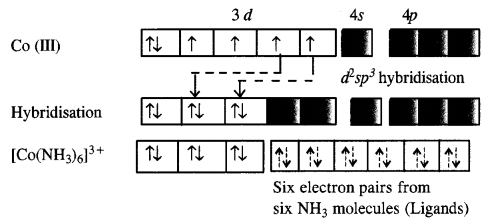As inner d-electrons are involved, the complex is inner orbital complex and is diamagnetic in nature.
In the complex [Ni(NH3)6]3 + , the oxidation state of Ni is +2 and has 3d8 configuration. Since six NH3 molecules (ligands) are to be accomodated, the hybridisation of metal ion is sp3d2. This means that 4d orbitals are involved in the hybridisation.The complex is paramagnetic as well as outer orbital complex since outer (4d) electrons are involved in the hybridisation.

Question 9.
Predict the number of unpaired electrons in the square planar [Pt(CN)4]2- ion.
The element Pt(Z = 78) is present in group 10 with electronic configuration 5d96s1. The divalent cation Pt2+ has 5d8 configuration.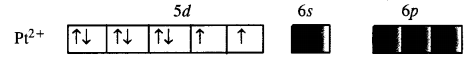For square planar complex, Pt (II) is in dsp2 hybridisation state. To achieve this, the two unpaired electrons present in 5d orbitals get paired. The complex has, therefore, no unpaired electrons.

Question 10.
The hexaquomanganese(II) ion contains five unpaired electrons, while the hexacyano ion contains only one unpaired electron. Explain using Crystal Field Theory.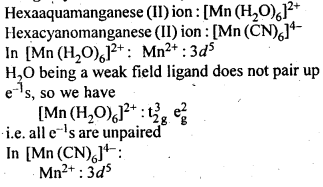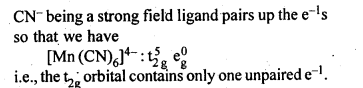Question 11.
Calculate the overall complex dissociation equilibrium constant for [Cu(NH3)4]2+ ion, given that p4 for the complex is 2·1 x 1013.
The dissociation constant is the reciprocal of overall stability constant (β4)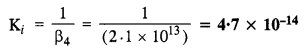NCERT EXERCISE

Question 1.
Explain bonding in co-ordination compounds in terms of Werner’s postulates.
Werner’s coordination theory: Alfred Werner gave his co-ordination theory in 1893. The important postulates of this theory are:
(i) All metals in atomic or ionic form exhibit two types of valencies in coordination compounds :
(a) Primary or principal or ionic valency (—–),
(b) Secondary or auxiliary or nonionic valency (—).
The primary valency is ionizable and it is shown by dotted lines. The secondary valency is non-ionizable and is shown by a continuous line.
(ii) Primary valency represents oxidation states of a metal atom or ion and secondary valency represents the co-ordination number of metal ion which is fixed for a particular atom.
(iii) The primary valencies are satisfied by negative ions whereas the secondary valencies may be satisfied either by negative ions (e.g., Cl, Br, CN etc.) or neutral molecules (ag., H2O).
(iv) Secondary valencies are directed towards a fixed position in space.
(v) Every element tends to satisfy both its primary and secondary valencies. For this purpose a negative ion may often act a dual behaviour i.e., it may satisfy primary as well as secondary valency (—–,-).
Example : (i) Luteo cobaltic chloride CoCl3.6NH3 or [Co(NH3)6]Cl3.
(ii) Purpureo cobaltic chloride COC13.5NH3 or [Co(NH3)5Cl]Cl2

Question 2.
FeSO4 solution mixed with (NH4)2SO4solution in 1:1 molar ratio gives the test of, Fe2+ion but CuSO4solution mixed with aqueous ammonia in 1:4 molar ratio does not give the test of Cu2+ ion. Explain why.
FeSO4 solution mixed with (NH4),SO4 solution in 1 : 1 molar ratio forms a double salt, FeS04 (NH4)2SO4-6H2O (Mohr’s salt) which ionizes in the solution to give Fe2+ions. Hence it gives the tests of Fe2+ ions. CuSO4 solution mixed with aqueous ammonia in 1:4 molar ratio forms a complex salt, with the formula [CU(NH3)4]SO4. The complex ion [Cu(NH3)4]2+ does not ionize to give Cu2+ ions. Hence, it does not give the tests of Cu2+ ion.

Question 3.
Explain with two examples each of the following:
coordination entity, ligand, coordination number, coordination polyhedron, homoleptic and heterolepic.
(a) Co-ordination Entity: A coordination complex or entity is the species enclosed in square bracket. It is also called co-ordinate sphere. It contains in it a certain metal atom or ion to which a fixed number of neutral molecules or ions capable of donating electron pairs are linked with co-ordinate bonds. e.g. [COCl3(NH3)3]

(b) Ligand: Ligands are the electron donor molecules or ions which may be either neutral, or anionic (sometimes cationic as well) and are linked to the central metal atom or ion by co-ordinate bonds also called dative bonds. In fact, the electrons needed for the bond are provided by the ligands. e.g. H2NCH2CH2NH2 or N(CH2CH2NH2)3

(c) Co-ordination Number: The coordination number [C.N.] of a metal ion in a complex can be defined as the number of ligand or donor atoms to which the metal is directly bonded. For example, in the complex ions, [PtCl6]2- and [Ni(NH3)4]2+, the coordination number of Pt and Ni are 6 and 4 respectively. Similarly, in the complex ions, [Fe(C2O4)3]3- and [CO(en)3]3+,the coordination number of both, Fe and Co, is 6 because C2O42-and en (ethane-1,2-diamine) are bidentate ligands.

(d) Coordination polyhedron : The spatial arrangement of the ligand atoms which are directly attached to the central atom/ion defines a coordination polyhedron about the central atom. The most common coordination polyhedra are octahedral, square planar and tetrahedral. For example, [CO(NH3)6]3+ is octahedral, [Ni(CO)4] is tetrahedral and [PtCl4]2- is square planar.

(e) Homoleptic and heteroleptic complexe : Complexes in which a metal is bound to only one kind of donor groups, e.g., [CO(NH3)6]3+, are known as homoleptic. Complexes in which a metal is bound to more than one kind of donor group, e.g., [CO(NH3)4Cl2]+, are known as heteroleptic.

Question 4.
What is meant by unidentate, didentate and ambidentate ligands ? Give two examples for each.
Unidentate ligands are those which bind to the metal ion through a single donor atom, e.g., Cl , H2O.

Bidentate ligands are those which bind to the metal ion through two donor atoms. e.g., ethane-1,2-diamine (H2NCH2CH2NH2), oxalate (C2O42-) ion.

Ambidentate ligands are those which can bind to metal ion through two different donor atoms, e.g., NO2 and SCN ion.

Question 5.
Specify the oxidation numbers of metals in the following co-ordination entities :
(a) [Co(H2O)(CN)(en2)]2+
(b) [PtCl4]2-
(c) [Cr(NH3)3Cl3]
(d) [CoBr2(en)2]+
(e) K3[Fe(CN)6].
(a) O.N. of Co : x + 0 + (-1) + 2(0)= + 2 or x = + 2+ 1 = + 3
(b) O.N. of Pt : x + 4 (-1) =-2 or x =-2 + 4 = + 2
(c) O.N. of Cr : x + 3(0) + 3(- 1) = 0 or x = + 3
(d) O.N. of Co : x + 2(- 1) + 2(0) = + 1 or x = + 1 + 2 = + 3
(e) O.N. of Fe : x + 6 (- 1) = – 3 or x = – 3 + 6 = + 3

Question 6.
Using IUPAC norms write the formulas for the following:
(i)Tetrahydroxozincate(Il)
(iii)Diamminedichlorido platinum (II)
(iv)Potassium tetracyanonickelate (II)
(v)Pentaamminenitrito-O-cobalt(III)
(vi)Ilexaamminccobalt (III) sulphate
(vii)Potassium tri(oxalato) chromate (III)
(yiii)Hexaammineplatinum (IV)
(ix)Tetrabromidocuprate(II)
(x) Pentaamminenitrito-N-cobalt (III)
(i)[Zn(OH)4]2- (ii)K2[PdCl4]
(iii)[Pt(NH3)2Cl2]
(iv)K2[Ni(CN)4]
(v)[Co(NH3)5(ONO)]2+
(vi)[Co(NH3)6]2(SO4)3
(vii)K3[Cr(C2O4)3]
(viii)[Pt(NH3)6]4+
(ix)[CuBr4]2-
(x)[Co(NH3)5(N02)]2+

Question 7.
Using IUPAC norms write the systematic names of the following :
(a) [CO(NH3)6]Cl3
(b) [CO(NH3)4Cl(NO2)]Cl
(c) [Ni(NH3)6]Cl2
(d) [Pt(NH3)2Cl(NH2CH3)]Cl
(e) [Mn(H2O)6]2+
(f) [NiCl4]2-
(g) [Co(en)3]3+
(h) [Ti(H2O)6]3+
(i) [Ni(CO)4]. (Jharkhand Board 2015)
(a) hexamminecobalt(III) chloride
(b) tetramminechloriodonitrito-N-cobalt(III) chloride
(c) hexaamminenickel(II) chloride
(d) diamminechlorido (methaneamine) platinum(II) chloride
(e) hexaaquamanganese(II) ion
(f) tetrachloriodonickelate(II) ion
(g) tris(ethane-l, 2-diammine) cobalt(III) ion
(h) hexaaquatitanium(III) ion
(i) tetracarbonylnickel (0).

Question 8.
List various types of isomerism possible for coordination compounds, giving an example of each.
(i) Geometrical isomerism: The isomer in which similar ligands occupy adjacent positions is referred to as cis isomer and the isomer in which similar ligands occupy opposite positions is referred to as trans isomer. Therefore, this type of isomerism is also known as cis-trans isomerism.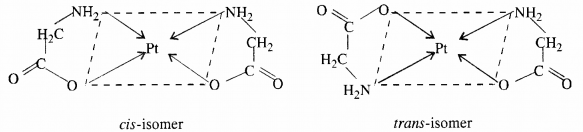(ii) Optical isomerism: The isomer which rotates the plane of polarised light to the right is called dextro rotatory and designated as d- and the one which rotates the plane of polarised light to the left is called laevo rotatory and designated as l. These optical isomers have identical physical and chemical properties except their behaviour towards the plane polarised light.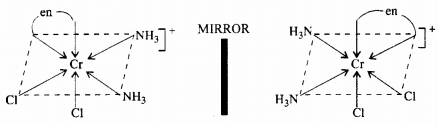(iii) Linkage isomerism: Linkage isomerism arises in a coordination compound containing ambidentate ligand. A simple example is provided by complexes containing the thiocyanate ligand, NCS, which may bind through the nitrogen to give M-N CS or through sulphur to give M-SCN. This behaviour was seen in the complex [CO(NH3)5(NO2)]Cl2, which is obtained as the red form, in which the nitrite ligand is bound through oxygen ( ONO), and as the yellow form, in which the nitrite ligand is bound through nitrogen (-NO2).

(iv) Coordination isomerism : This type of isomerism arises from the interchange of ligands between cationic and anionic entities of different metal ions present in a complex. An example is provided by [Co(NH3)6] [Cr(CN)6], in which the NH3 ligands are bound to CO3+ and the CN ligands to Cr3+. In its coordination isomer [Cr(NH3)6][CO(CN)6], the NH3 ligands are bound to Cr3+ and the CN ligands to CO3+.

(v) Ionisation isomerism : This form of isomerism arises when the counter ion in a complex salt is itself a potential ligand and can displace a ligand which can then become the counter ion. An example is provided by the ionisation isomers [CO(NH3)5SO4]Br and [CO(NH3)5Br]SO4.

(vi) Solvate isomerism : This form of isomerism is known as ‘hydrate isomerism in case where water is involved as a solvent. This is similar to ionisation isomerism. Solvate isomers differ by whether or not a solvent molecule is directly bonded to the metal ion or merely present as free solvent molecules in the crystal lattice. An example is provided by the aqua complex [Cr(H2O)6]Cl3 (violet) and its solvate isomer [Cr(H2O)5Cl]Cl2 H2O (grey green).

Question 9.
How many geometrical isomers are possible in the following coordination entities ?
(a) [Cr(C2O4)3]3-
(b) [Co(NH3)3Cl3]
(a) [Cr(C2O4)2]3- : No geometrical isomerism is possible.
(b) [CO(NH3)3Cl3] : Two geometrical isomers : fac and mer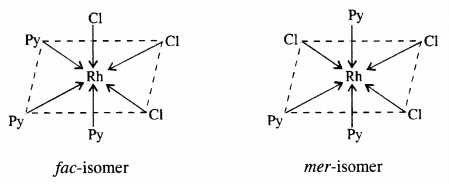Question 10.
Draw the structures of optical isomers of :
(a) [Cr(C2O4)3]3-
(b) [PtCl2(en)2]2+
(c) [Cr(NH3)2Cl2(en)]+ (C.B.S.E. Foreign 2015)Question 11.
Draw all the isomers (geometrical and optical) of :
(a) [CoCl2(en)2]+
(b) [CoNH3Cl(en)2]2+
(c) [Co(NH3)2Cl2(en)]+
(a)(b)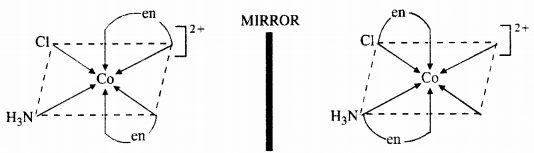(c)Question 12.
Write all the geometrical isomers of [Pt(NH3)(Br)(Cl)(py)]. How many of these will exhibit optical isomerism ?
There are three geometrical isomers.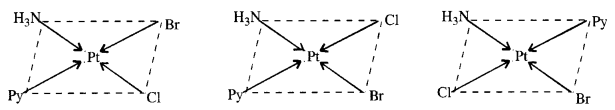Optical isomerism is generally not shown by the square planar complexes with CN = 4.

Question 13.
Aqueous copper sulphate solution (blue in colour) gives (a) green precipitate with aqueous potassium fluoride and (b) a bright green solution with aqueous potassium chloride solutions. Explain these experimental results.
Aqueous solution of copper sulphate which is blue in colour exists as [Cu(H2O)4]SO4 and gives [Cu(H2O)4]2+ in solution. It is a labile complex entity in which the ligands H2O get easily replaced by F ions of KF and by Cl ions of KCl.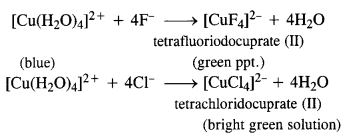Question 14.
What is the coordination entity formed when excess aqueous KCN is added to an aqueous solution of copper sulphate? Why is that no precipitate of copper sulphide is obtained when H2S(g) is passed through the solution?
When an excess aqueous KCN is added to an aqueous solution of CuSO4, Potassiumtetra-cyanocuprate (II) is formed. When H2S(g) is passed through the above solution, no precipitate of copper sulphide is obtained because CN ions are strong ligands so the complex [Cu(CN)4]2- is very stable. As Cu2+ ions are not available so CuS precipitate is not formed.Question 15.
Discuss the nature of bonding in the following co-ordination complexes on the basis of valence bond theory :
(a) [Fe(CN)6]4-
(b) [FeF6]3-
(c) [Co(C2O4)3]3-
(d) [CoF6]3-
(a) Hexacyanoferrate(II) ion [Fe(CN)6]4-: Iron in this complex is in +2 oxidation state. Iron achieves + 2 oxidation state by the loss of two 4s electrons. The resulting Fe2+ ion has outer electronic configuration of 3d6.(b) Hexafluoriodoferrate(II) ion [FeF6]3-: Iron in this complex is in +2 oxidation state. Iron achieves + 2 oxidation state by the loss of two 4s electrons. The resulting Fe2+ ion has outer electronic configuration of 3d6.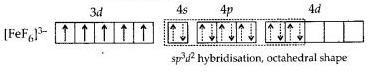(c)(d) Hexafluorocobaltate(III) [CoF6]3-: Cobalt ion in the complex is in + 3 oxidation state. Cobalt achieves + 3 oxidation state by the loss of two 4s electrons and one 3d electron. The resulting Co3 + ion has outer electronic configuration of 3d6.Question 16.
Draw figure to show the splitting of d-orbitals in octahedral crystal field.
Let us assume that the six ligands are positioned symmetrically along the Cartesian axes, with a metal atom at the origin.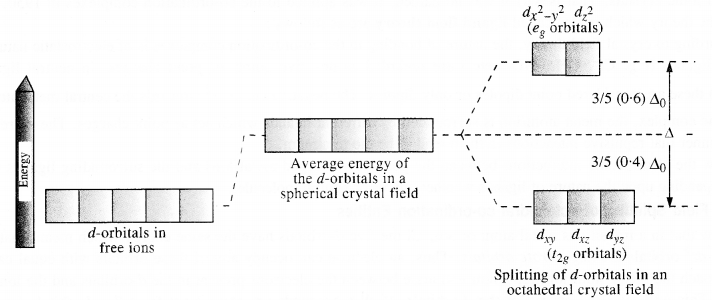As the ligands approach, first there is an increase in energy of d-orbitals relative to that of the free ion just as would be the case in a spherical field.

The orbitals lying along the axes (dz2 and dx2 – y2) get repelled more strongly than dxy, dyz and dzx orbitals which have lobes directed between the axes.

The dz2 and dx2-y2 orbitals get raised in energy and dxy dyz, dxz orbitals are lowered in energy relative to the average energy in the spherical crystal field. Thus, the degenerate set of d-orbitals get split into two sets: the lower energy orbitals set, t2g, and the higher energy orbitals set, eg. The energy is separated by ∆0

Question 17.
What is spectrochemical series? Explain the difference between a weak field ligand and a strong field ligand.
Spectrochemical series: The arrangement of ligands in order of their increasing field strengths i.e. increasing crystal field splitting energy (CFSE) values is called spectrochemical series, which is as follows:
I < Br < SCN < Cl < S2- < F < OH < C2O2-4 < H2O < NCS < edta-4 < NH3 < en < CN < CO.
Difference between weak field ligand and a strong field ligand: The ligand with a small value of CFSE (∆0) are called weak field ligands whereas those with a large value of CFSE are called strong field ligands.

Question 18.
What is crystal field splitting energy ? How does the magnitude of ∆0 decide the actual configuration of d- orbitals in a coordination entity ?
The degenerate d-orbitals (in a spherical field environment) split two-level i.e. eg and t2g in the presence of ligands. The splitting of the degenerate orbitals in the presence of ligands is called crystal field splitting and the energy difference between the two levels (e and t2g) is called the crystal field splitting energy. It is denoted by ∆o. After the orbitals have split, the filling of the electrons takes place. After 1 electron (each) has filled in the three t2g orbitals, the filling of the electrons takes place in 2 ways.

It can enter the orbital (giving) rise to t3g eg, like electronic configuration on the pairing of the electrons can take place in the t2g orbitals (giving rise to t42g eg0 like electronic configuration). If the ∆o value of a ligand is less than the pairing energy, then the electrons enter the eg orbital. On the other hand, if the ∆o value of a ligand is more than the pairing energy, then the electrons enter the t2g orbitals.

Question 19.
[Cr(NH3)6]3+ is paramagnetic while [Ni(CN)4]2- is diamagnetic. Explain why?
(i) Hexaamminechromium(III) ion [Cr(NH3)6]3+: Outer electronic configuration of chromium (Z=24) in ground state is 3d24s1 and in this complex, it is in the +3 oxidation state. Chromium achieves +3 oxidation state by the loss of one 4s electron and two 3d-electrons. The resulting Cr3+ ion has outer electronic configuration of 3d3.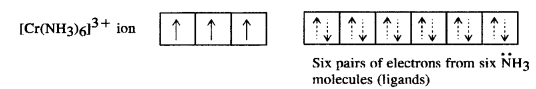(ii) Tetracyanonickelate (II) ion [Ni(CN)4]2-: Outer electronic configuration of nickel (Z = 28) in ground state is 3d84s2. Nickel in this complex is in + 2 oxidation state. It achieves + 2 oxidation state by the loss of the two 4s-electrons. The resulting Ni2+ ion has outer electronic configuration of 3d8.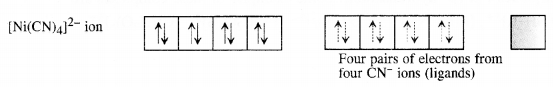Question 20.
A solution of [Ni(H2O)6]2+ is green but a solution of [Ni(CN)4]2- is colourless. Explain. (C.B.S.E. Delhi 2017)
Formation of [Ni(H2O)6]2+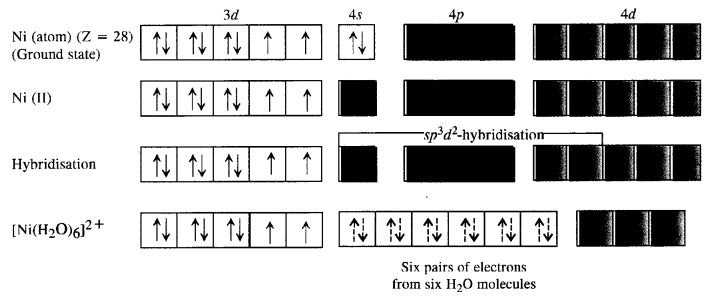Since H2P molecules represent weak field ligands, they do not cause any electron pairing. As a result the complex has two unpaired electrons and is coloured. The d-d transitions absorb radiations corresponding to a red light and the complementary colour emitted is green.
Formation of [Ni(CN)4]2-. For the details of the structure of the complex,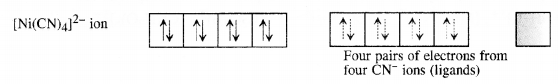Since the complex has no unpaired electrons there is no scope for any d-d transition. The complex is therefore, colourless.

Question 21.
[Fe(CN)6]4- and [Fe(H2O)6]2+ are of different cdlours in dilute solutions. Why?
In both the complexes, Fe is in +2 state with the configuration 3d6 i.e., it has four unpaired electrons. As the ligand H2O and CN possess different crystal field splitting energy (∆0), they absorb different components of the visible light (VIBGYOR) for the transition. Hence, the transmitted colours are different.

Question 22.
Discuss the nature of bonding in metal carbonyls.
The metal-carbon bond in metal carbonyls possess both s and p character. The M-C σ bond is formed by the donation of lone pair of electrons on the carbonyl carbon into a vacant orbital of the metal. The M-C π bond is formed by the donation of a pair of electrons from a filled d-orbital of metal into the vacant antibonding π* orbital of carbon monoxide. The metal to ligand bonding creates a synergic effect which strengthens the bond between CO and the metal.Question 23.
Give the oxidation state, d-orbital occupation and coordination number of the central metal ion in the following complexes :
(a) K3[CO(C2O4)3]
(b) (NH4)2[CoF4]
(C) Cis – [CrCl22(en)2]Cl
(d) [Mn(H2O)6]SO4
(a) OS = + 3, CN = 6, d-orbital occupation is 3d6 $${ t }_{ 2g }^{ 6 }{ e }_{ g }^{ 0 }$$,
(b) OS = + 2, CN = 4, 3d7 ($${ t }_{ 2g }^{ 5 }{ e }_{ g }^{ 2 }$$),
(c) OS = + 3, CN = 6, 3d3 ($${ t }_{ 2g }^{ 3 }$$),
(d) OS = + 2, CN = 6, 3d6 ($${ t }_{ 2g }^{ 3 }{ e }_{ g }^{ 2 }$$).

Question 24.
Write down the IUPAC name for each of the following complexes and indicate the oxidation state, electronic configuration and coordination number. Also give stereochemistry and magnetic moment of the complex :
(a) K[Cr(H2O)2(C2O4)2]3H2O
(b) [CrCl3(py)3]
(c) K4[Mn(CN)6]
(d) [Co(NH3)5Cl]Cl2
(e) Cs[FeCl4]
(a) IUPAC name : potassium diaquadioxalatochromate (III) hydrate.
O.S. of Cr = + 3 ; 3d3 ($${ t }_{ 2g }^{ 3 }{ e }_{ g }^{ 0 }$$) CN = 6 ; shape = octahedral, three unpaired electrons.
Magnetic moment (μ) = $$\sqrt { n\left( n+2 \right) } =\sqrt { 3\times 5 } =\sqrt { 15 } =3\cdot 87BM$$
(b) IUPAC name: trichloridotripyridinechromium (III) O.S. of Cr = + 3; 3 d3 ($${ t }_{ 2g }^{ 3 }{ e }_{ g }^{ 0 }$$) CN = 6
shape = octahedral ; three unpaired electrons.
Magnetic moment (μ) = $$\sqrt { n\left( n+2 \right) } =\sqrt { 3\times 5 } =3\cdot 87BM$$
(c) IUPAC name : potassiumhexacyanomanganate (II) O.S. of Mn = + 2 ; 3d5 ($${ t }_{ 2g }^{ 5 }{ e }_{ g }^{ 0 }$$), CN = 6, shape = octahedral; one unpaired electron.
Magnetic moment (μ) = $$\sqrt { n\left( n+2 \right) } =\sqrt { 1\times 3 } =\sqrt { 3 } =1\cdot 73BM$$
(d) IUPAC name : pentaamminechloridocobalt (III) chloride
O.S. of Co = + 3 ; 3d6 ($${ t }_{ 2g }^{ 6 }{ e }_{ g }^{ 0 }$$), CN = 6
shape = octahedral; zero unpaired electron. Magnetic moment (μ) = 0
(e) IUPAC name : cesium tetrachloridoferrate (III)
O.S. of Fe = + 3 ; 3d5 ($${ e }^{ 2 }{ t }_{ 2 }^{ 3 }$$), CN = 4.
shape = tetrahedral ; five unpaired electrons.
Magnetic moment (μ) = $$\sqrt { n\left( n+2 \right) } =\sqrt { 5\times 7 } =\sqrt { 35 } =5\cdot 92BM$$

Question 25.
What is meant by stability of a coordination compound in solution ? State the factors which govern stability of complexes.
The stability of a complex in solution refers to the degree of association between the two species involved in the state of equilibrium. The magnitude of the (stability or formation) equilibrium constant for the association, quantitatively expresses the stability. Thus, if we have a reaction of the type :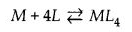then, the larger the stability constant, the higher is the proportion of ML4 that exists in the solution. Free metal ions rarely exist in the solution so that M will usually be surrounded by solvent molecules which will compete with the ligand molecules, L, and be successively replaced by them. For simplicity, we generally ignore these solvent molecules and write four stability constants as follows :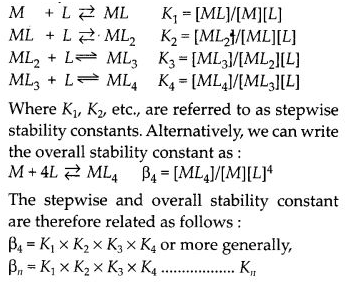Factors affecting stability of complexes :

1. The smaller the size of the cation, the greater will be the stability of the complex e.g., Fe3+ forms a more stable complex than Fe2+.
2. The greater the charge on the central metal ion, the more stable will be the complex e.g., Pt4+ forms a more stable complex than Pt2+.
3. Stronger the ligand, more stable will be the complex formed e.g., CN forms more stable complex then NH3.

Question 26.
What is meant by chelate effect ? Give an example.
When a ligand attaches to the metal ion in a manner that form’s a ring, then the metal-ligand association is found to be more stable. In other words, we can say that complexes containing chelate ring more stable than complexes without rings. This is known as the chelate effect.
Examples: EDTA, DMG, etc.

Question 27.
Discuss briefly giving an example in each case the role of coordination compounds in:
(i) biological systems
(ii) medicinal chemistry
(iii) analytical chemistry and
(iv) extraction/ metallurgy of metals.
(i) Role of coordination compounds in biological systems:
We know that photosynthesis is possible by the presence of chlorophyll pigment. This pigment is a coordination compound of magnesium. In the human biological system, several coordination compounds play important roles. For example, the oxygen – carrier of blood, i.e hemoglobin is a coordination compound of iron.

(ii) Role of coordination compounds in Medicinal chemistry: Certain coordination compounds of platinum (for example cis-platin) are used for inhibiting the growth of tumors.

(iii) Role of coordination compounds in analytical chemistry: During salt analysis, a number of basic radicals are detected with the help of the colour changes they exhibit with different reagents. These colour changes are a result of the coordination compounds or complexes that the basic radicals form with different ligands.

(iv) Role of coordination compounds in interaction or metallurgy of metals: The process of extraction of some of the metals from their ores involves the formation of complexes. For example in an aqueous solution, gold combines with cyanide ions to form [Au (CN)2]. From this solution, gold is later extracted by the addition of Zn metal.

Question 28.
How many ions are produced from the complex Co(NH3)6Cl2 in solution?
(a) 6
(b) 4
(c) 3
(d) 2.
The complex will dissociate in aqueous solution to give three ions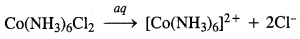Therefore, (c) is the correct answer.

Question 29.
Amongst the following ions which one has the highest magnetic movement value?
(a) [Cr(H2O)6]3+
(b) [Fe(H2O)6]2+
(c) [Zn(H2O)6]2+
The oxidation states of the metals in the complexes along with the electronic configuration are given:
(a) Cr3+ : 3d3 configuration ; unpaired electrons = 3
(b) Fe2+ : 3d6 configuration ; unpaired electrons = 4
(c) Zn2+ : 3d10 configuration ; unpaired electrons = 0
The complex (b) with maximum number of unpaired electrons has the highest magnetic moment. Therefore, (b) is the correct answer.

Question 30.
The oxidation number of cobalt in K[Co(CO)4] is
(a) + 1
(b) + 3
(c) – 1
(d) – 3.
O.N. of Co : x + 4(0) = -1 or x = -1. Therefore, (c) is the correct answer.

Question 31.
Amongst the following, the most stable complex is :
(a) [Fe(H2O)6]3+
(b) [Fe(NH3)6]3+
(c) [Fe(C2O4)3]3-
(d) [FeCl6]3-.
In all the complexes, Fe is in + 3 oxidation state. However, the complex (c) is a chelate because three $${ C }_{ 2 }{ O }_{ 4 }^{ 2- }$$ ions act as the chelating ligands. Thus, the most stable complex is (c).
H2O < NH3 < $${ NO }_{ 2 }^{ – }$$